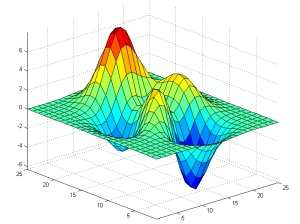M442:Numerical Solution of Differential EquationsInstructor Mark Pernarowski Textbook Numerical Mathematics and Computing, 7th edition Cheney and Kincaid Office Hours Schedule (Wil 2-236) Phone 994-5356 Classroom Wil 1-144` Grading: The course % for M442 is determined by:   Midterm       M            100    Final         F            100   Homework      HW           200  _______________________________                              400          % = (M+F+HW)/4 The final is not comprehensive and both the final and midterm are take home exams. Homework and exam due dates will be announced in class and posted here at a later date. Exam content will be announced in class. Midterm and Final due dates and details will be announced in class.` ` Homework: Assigned homework will be posted below as the course develops. Homework scores will vary depending on their length and difficulty. The raw scores will be summed, and converted into a % to yield the 200 points in the final grade.Computing: Some assignemnts will require the use of matlab. As students, you are entitled to free use of this software. You will eitherdownload it to your computer or may use it on campus computers in the library where it is installed.``Handouts: PDF handouts will eitherbe emailed to you or handed out inclass (hardcopy)`Rough Syllabus: (select topics from the chapters listed below)

1. Chapter 4: Interpolation and Numerical Differentiation
2. Chapter 5: Numerical Integration

3. Chapter 7: Initial Value Problems
4. Chapter 11: Boundary Value Problems

5. Chapter 12: Partial Differential Equations

Attendance in class is paramount to knowing which subtopics of each chapter will be covered!

In class I may cover the topics in a manner different from the textbook.Matlab code for M442:

 interp.m phi.m Code to interpolate data X with functions phi(x,n) ld.m Code declaring cardinal polynomials as a function ld(i,x,X), where X=data x values cardinal.m Code for polynomial interpolation using cardinal polynomials in ld.m

Matlab code for M441:

 Df_procedure.m secant approximation for derivative of sin(x) Df.m Df_procedure declared as a function Horner.m Method for fast polynomial evaluation f.m a function for Bisect.m g.m a different function for Bisect.m Bisect.m Bisection Method BisectF.m Bisection Method: fancy version f.m a function for Newton.m df.m the derivative of f(x) in f.m Newton.m Newton's method NewtonPlot.m plots tangent lines in Newton method. Secant.m Secant Method GaussNaive.m Gauss step by step solver Elementary.m Elementary matrices and LU decomp. Needs this too: Elem.m phi.m Power Method Selector function Power.m Power method example Iterate.m Richardson, Jacobi, Gaus-Seidel, SOR iterative methodsgetting matlab a link to downloading matlab on MSU campus. You will need intro of campus link with simple introduction to syntax in matlab mathworks company that develops and maintains matlabSchedule HW due dates and Exam Due dates will be posted here as the course develops

 Sunday Monday Tuesday Wed Thursday Friday Saturday 12 13Classes start 14 15 16 17 18 19 20MLK Day 21 22 23 24 25 26 27 28 29 30 31 1 2 3 4 5 6 7 8 9 10 11 12 13 14 15 16 17 Pres. Day 18 19 20 21 22 23 24 25 26 27 28 29 1 2 3 4 5 6 7 8 9 10 11 12 13 14 15 16Spring Break 17Spring Break 18Spring Break 19Spring Break 20Spring Break 21 22 23 24 25 26NCUR 27 NCUR 28 29 30 31 1 2 3 4 5 6 7 8 9 10Univer. Day 11 12 13 14 15 16 17 18 19 20 21 22 23 24 25 26 27 28 29 30 1 Classes End 2 3 4 Final12-2pm 5 6 7 8 9